# Boolean Algebra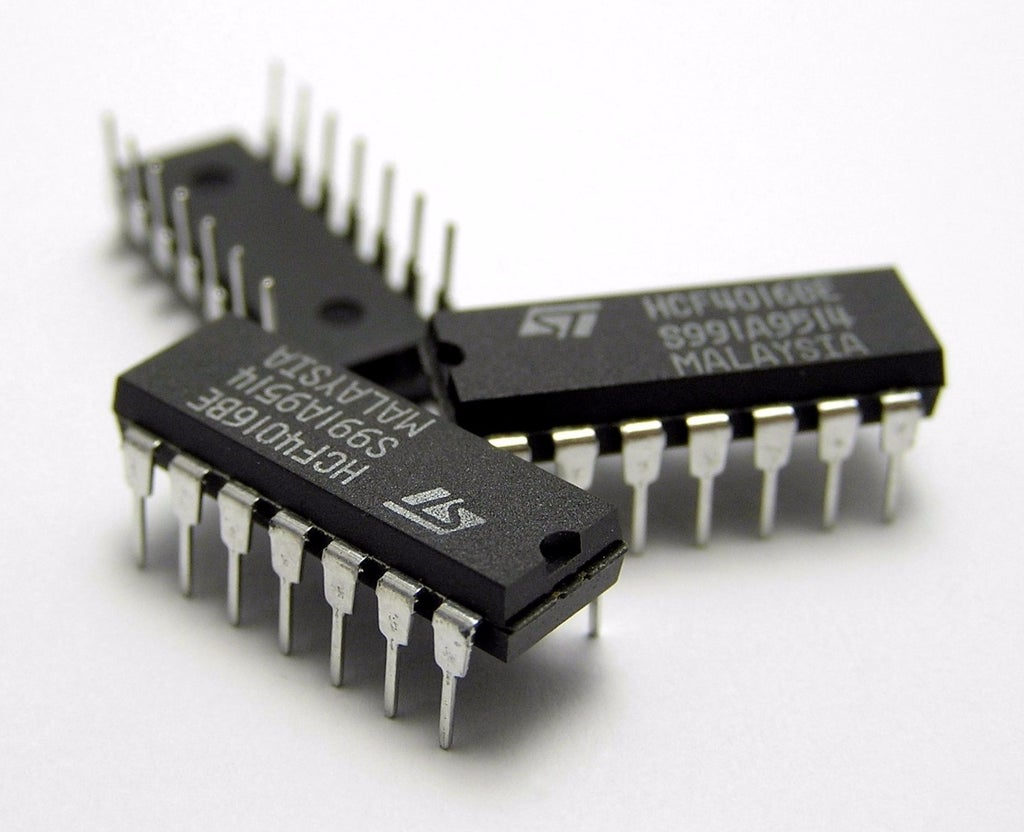Written by

We are all familiar I guess with the basic logic gates and know what they do and how to use them. Large systems built from logic gates used to be common, although they have mostly been replaced by microcontrollers or FPGAs these days. Nevertheless, logic still has a place in modern circuit design, especially in “glue logic”—those small pieces of logic that connect various functions in our circuits.

You can still buy logic in the classic 7400 or 4000 series gates updated for the lower voltage levels we use today, but the manufacturers have recognized the changing role of logic and introduced a range of smaller logic gates consisting of just a single gate or a couple of gates in a single package. Such examples include TI’s “Little Logic”, Diodes Inc’s “Single Gate” and “Dual Gate” family and many others. These families include configurable gates, and some include level translation as well which can be very handy.

Optimizing your logic to use the minimum number of gates (or use the ones that you have in inventory) is much easier if you know a bit of Boolean algebra. This is the algebra we get when a variable an only have the values of “true” or “false” which we represent as a 1 or 0 respectively.

There are three basic operators corresponding to the three basic logic gate types—AND, OR and NOT. We use the + symbol to indicate the OR operator so we write “a OR b” as a + b. We use the dot operator to indicate the AND operator, so we write “a AND b” as a · b. Finally, we use the overbar to indicate the NOT or inversion operator, so we write “a AND NOT b” as a · b̅. We can use parentheses to specify the order of operations just as we do with conventional algebra.

Before we move on, we should mention the exclusive-or (XOR) operator. This is not a basic function, since it can be derived from the basic operators, but it occurs often enough that it gets a special symbol of its own. We write “a XOR b” as a ^ b.

And just like regular algebra there are a series of laws which allow us to expand expressions, collect like terms and otherwise manipulate our logic expression to get it into the optimum form for implementation. Figure 1 shows a list of these laws – most are similar to conventional algebra if you think of the OR operator producing a sum and the AND operator producing a product.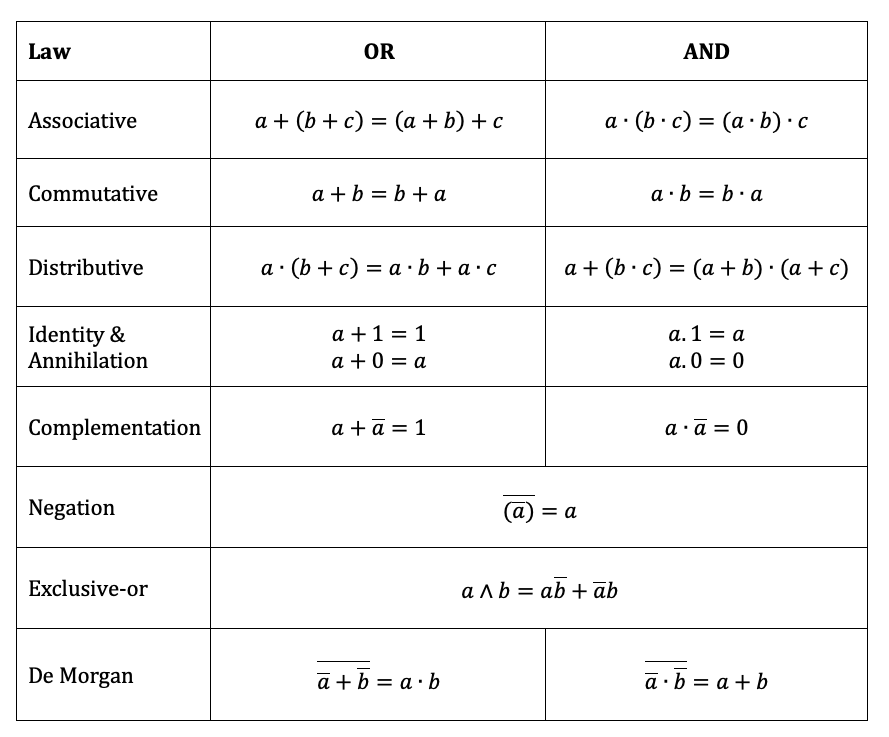FIGURE 1. The first three laws of Boolean algebra are very similar to the laws of regular algebra if you think of the OR operator producing a sum and the AND operator producing a product. The rest of the laws are applicable only to Boolean algebra. De Morgan’s theorem is especially useful as it allows us to swap AND and OR operators with a bit of negation.

One of the key laws is De Morgan’s theorem which is specific to Boolean algebra.  This allows us to swap between an AND relationship to an OR relationship with the help of some inversion. It may help to look at this in terms of gates as shown in Figure 2.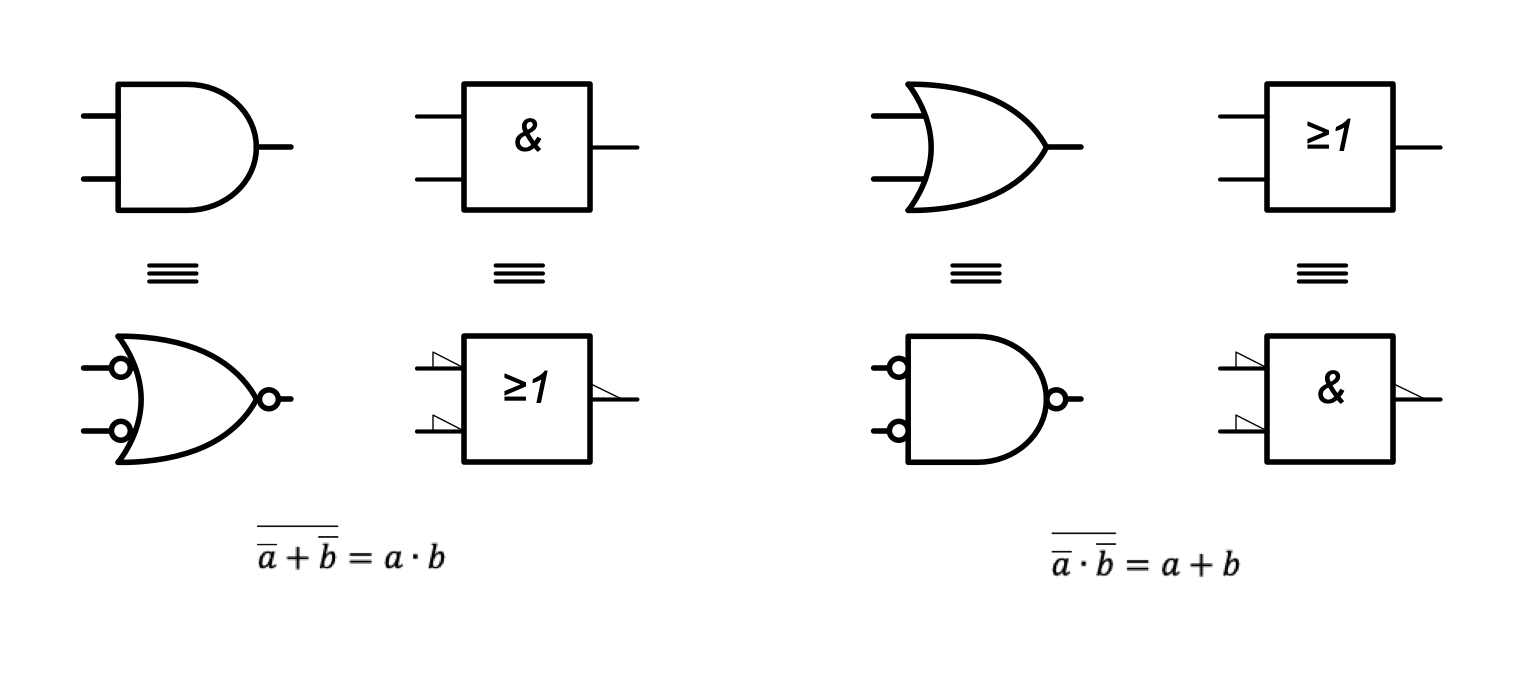FIGURE 2. This figure shows the De Morgan equivalent of the AND and OR gates. The circles or triangles on the input and output of the gates represent negation. The IEEE symbols on the left are commonly used in the USA and the IEC symbols on the right are commonly used in Europe.

Let’s work a (simple) real-life problem to illustrate the use of Boolean algebra. The Gray code is an ordering of normal binary code such that only one bit changes each count.  Figure 3 shows the first eight binary digits and their corresponding Gray codes.  Gray code counting is sometimes used in precision encoders because you can detect a missing count if more than one-bit changes between readings. Suppose we want to construct a logic circuit to convert a three-digit binary number to Gray code.FIGURE 3. This table shows the first eight binary numbers and their corresponding Gray code equivalents. The Gray code differs from binary in that only one bit changes between each successive count. This is useful because we can know we have missing counts if we see more than on bit change between readings.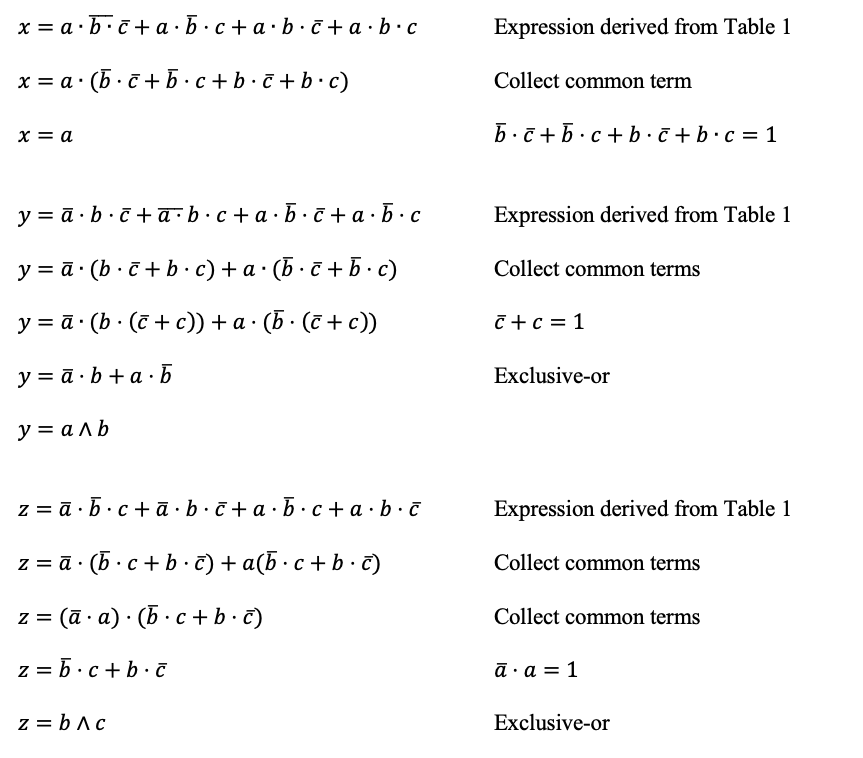FIGURE 4. This figure shows how the binary to Gray code converter expressions are simplified to implement the functionality shown in Figure 3. We start by collecting common terms and using identities to eliminate redundant variables. The result can be implemented with just two exclusive-or gates.

Figure 4 shows how we apply the Boolean logic laws to reduce the expressions. For each of the output bits x, y and z, we write down the logical expression by just reading off the table. We start simplification by collecting common terms and using identities to eliminate redundant variables. The expressions simplify considerably, and the resultant circuit as shown in Figure 5. The circuit requires just two exclusive-or gates and could be implemented with a single two-gate package such as a 74LVC2G86.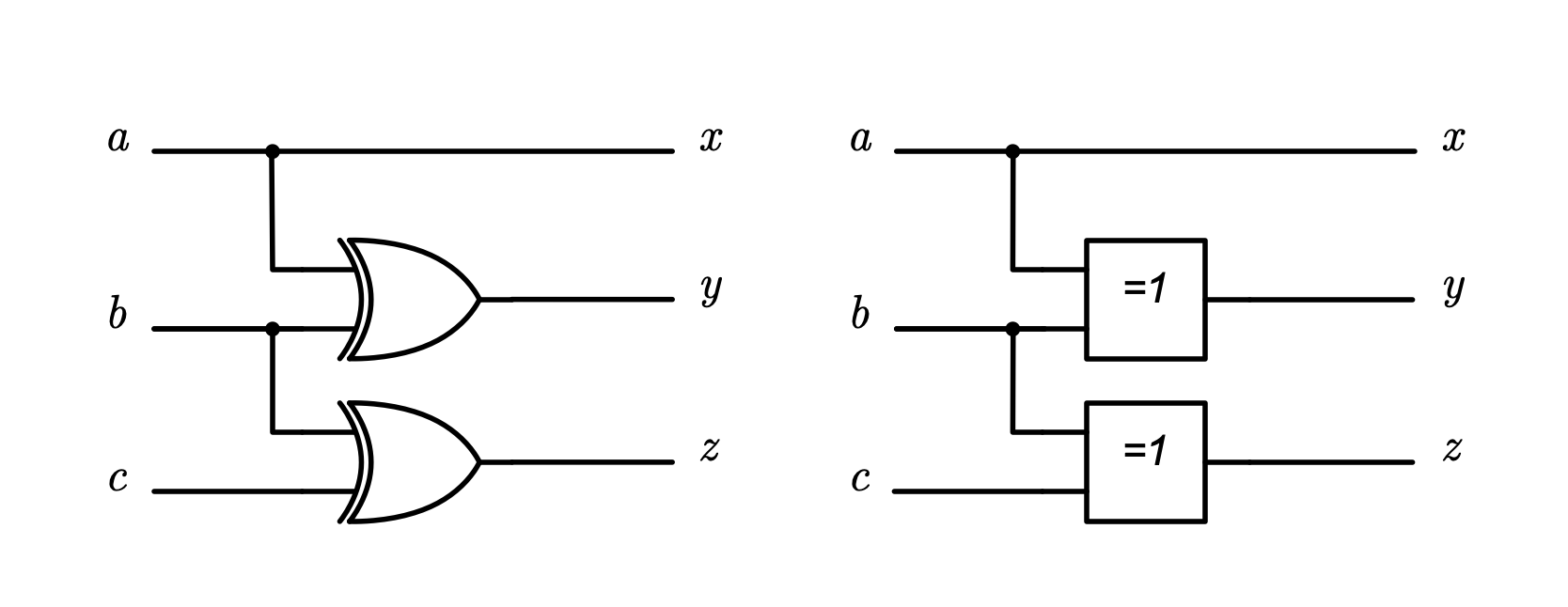FIGURE 5. This shows the binary to Gray code converter implemented in its simplest form. This could be implemented in a single two-gate package such as the 74LCV2G86. Both the IEEE and the IEC versions are shown.

References:
“Boolean Algebra.” In Wikipedia, January 2, 2022.
https://en.wikipedia.org/w/index.php?title=Boolean_algebra&oldid=1063362794.
Diodes. “Logic,” June 18, 2015. https://www.diodes.com/products/logic/

“Gray Code.” In Wikipedia, January 9, 2022.
https://en.wikipedia.org/w/index.php?title=Gray_code&oldid=1064629788.

 Keep up-to-date with our FREE Weekly Newsletter! Don't miss out on upcoming issues of Circuit Cellar. Subscribe to Circuit Cellar Magazine Note: We’ve made the May 2020 issue of Circuit Cellar available as a free sample issue. In it, you’ll find a rich variety of the kinds of articles and information that exemplify a typical issue of the current magazine. Would you like to write for Circuit Cellar? We are always accepting articles/posts from the technical community. Get in touch with us and let's discuss your ideas.